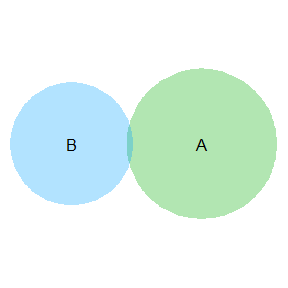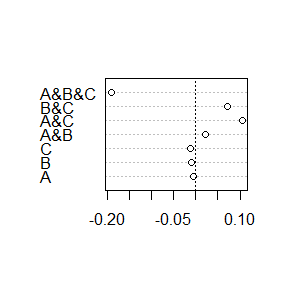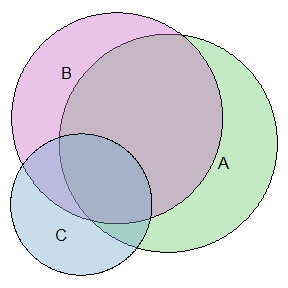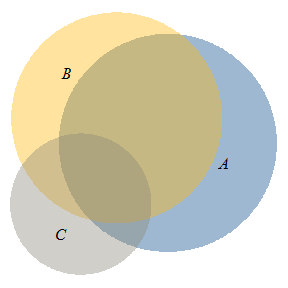# An introduction to eulerr

#### 2016-10-16

eulerr generates area-proportional euler diagrams that display set relationships (intersections, unions, and disjoints) with circles. Euler diagrams are Venn diagrams without the requirement that all set interactions be present (whether they are empty or not). That is, depending on input, eulerr will sometimes produce Venn diagrams but sometimes not.

## Background

R features a number of packages that produce euler and/or venn diagrams; some of the more prominent ones (on CRAN) are

The last of these serves as the primary inspiration for this package, along with the refinements that Ben Fredrickson has presented on his blog and made available in his javascript venn.js.

venneuler, however, is written in java, which prevents R users from browsing the source code (unless they are also literate in java) or contributing. Furthermore, venneuler is known to produce imperfect output for set relationships that have perfect euler diagram solutions. Consider, for instance

venn_fit <- venneuler::venneuler(c(A = 75, B = 50, "A&B" = 0))
par(mar = c(0, 0, 0, 0))
plot(venn_fit)that reasonably should not display any intersection between A and B.

## Enter eulerr

eulerr is based around the improvements to venneuler that Ben Fredrickson introcued with venn.js but with rewritten code, different optimizers, and methods to calculate stress statistics. It also provides a highly customizable interface for its plotting function.

### Input

Currently, it is possible to provide input to eulerr as either

• a named numeric vector or
• a matrix of logicals with columns representing sets and rows the set relationships for each observation.
library(eulerr)

# Input in the form of a named numeric vector
fit1 <- eulerr(c("A" = 25, "B" = 5, "C" = 5,
"A&B" = 5, "A&C" = 5, "B&C" = 3,
"A&B&C" = 3))

# Input as a matrix of logicals
set.seed(1)
mat <-
cbind(
A = sample(c(TRUE, TRUE, FALSE), size = 50, replace = TRUE),
B = sample(c(TRUE, FALSE), size = 50, replace = TRUE),
C = sample(c(TRUE, FALSE, FALSE, FALSE), size = 50, replace = TRUE)
)
fit2 <- eulerr(mat)

### Fit

We can expect our results by printing the eulerr object

fit2
## $coefficients ## x y r ## A 34.398248 19.1381437 33.09853 ## B 18.887664 26.7291801 32.01503 ## C 7.988108 0.5417698 21.44077 ## ##$original.values
##     A     B     C   A&B   A&C   B&C A&B&C
##    31    29    13    20     6     7     5
##
## $fitted.values ## A B C A&B A&C B&C A&B&C ## 31.005893 29.009133 13.010910 19.977026 5.894665 6.927797 5.191156 ## ##$residuals
##            A            B            C          A&B          A&C
## -0.005892980 -0.009132989 -0.010909949  0.022973583  0.105335028
##          B&C        A&B&C
##  0.072202508 -0.191155861
##
## $stress ##  2.941349e-05 ## ## attr(,"class") ##  "eulerr" "list" or directly access and plot the residuals and plot using standard methods. resid(fit2) ## A B C A&B A&C ## -0.005892980 -0.009132989 -0.010909949 0.022973583 0.105335028 ## B&C A&B&C ## 0.072202508 -0.191155861 # Cleveland dot plot of the residuals graphics::dotchart(resid(fit2)) abline(v = 0, lty = 3)This shows us that the A&B&C intersection is somewhat overrepresented in fit2. Althgouh, given that these residuals are on the scale of the original values, the residuals are arguably small. For an overall measure of the fit of the solution, we use the same stress statistic that Leland Wilkinson presented in his academic paper on venneuler (Wilkinson (2012)), which is given by the sums of squared residuals divided by the total sums of squares: $\frac{\sum \limits_{i=1}^n (f_i -y_i)^2}{\sum \limits_{i=1}^n (y_i - \bar{y})^2}$ For our solution, the stress is fit2$stress
##  2.941349e-05

, which is quite low.

We can now be confident that eulerr provides a reasonable representation of our input. Were it otherwise, we would do best to stop here and look for another way to visualize our data. (I suggest the excellent UpSetR package.)

### Plotting

No we get to the fun part: plotting our euler fit. This is easy, as well as highly customizable, with eulerr.

par(mar = c(0, 0, 0, 0))
plot(fit2)

# Change fill colors, border type (remove) and fontface.
plot(fit2,
polygon_args = list(col = c("dodgerblue4", "darkgoldenrod1", "cornsilk4"),
border = "transparent"),
text_args = list(font = 8))eulerr’s default color palette is taken from qualpalr – another package that I have developed – which uses color difference algorithms to generate distinct qualitative color palettes.

## Details

Details of the implementation will be left for a future vignette but almost completely resemble the approach documented here.

## Thanks

eulerr would not be possible without Ben Fredrickson’s work on venn.js or Leland Wilkinson’s venneuler.